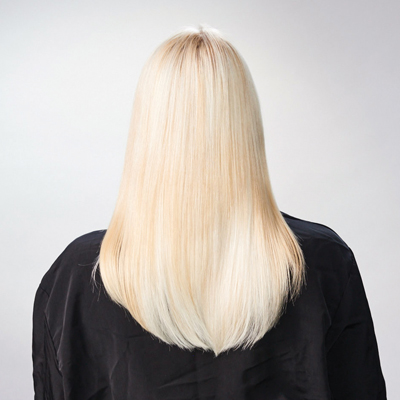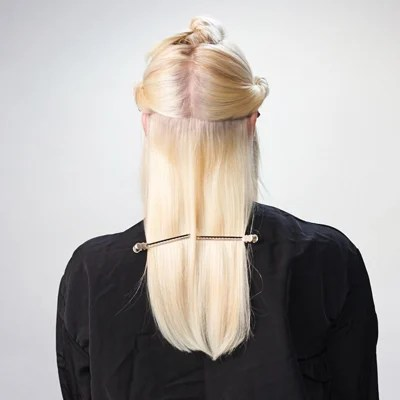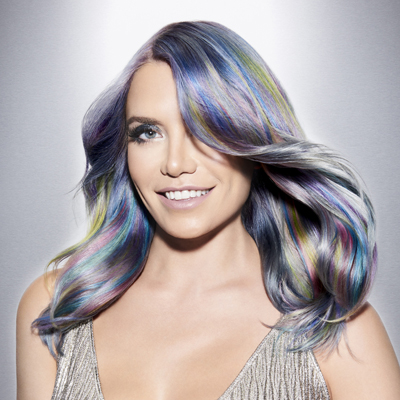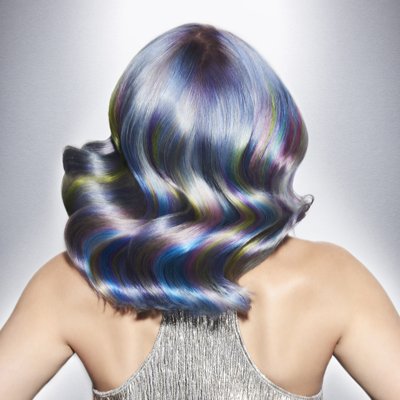Metallic Pop Art
January 29, 2017

# Metallic Pop Art

VIEW ALL PHOTOS VIEW SLIDESHOW
GET THE HOW-TO• Formula A

Joico Vero K-PAK VeroLight Lightening Powder + 10-volume Veroxide

• Formula B

Joico Vero K-PAK Lightening Powder + 20-volume Veroxide

• Formula C

Joico Vero K-PAK Color TSB + VeroGlaze No-Lift Crème Developer

• Formula D

Equal parts Joico Color Intensity Metallic Violet + Color Intensity Metallic Pewter

• Formula E

Equal parts Color Intensity Titanium + Color Intensity Metallic Pewter

• Formula F

Equal parts Color Intensity Mermaid Blue + Color Intensity Metallic Pewter

• Formula G

Equal parts Color Intensity Metallic Mauve Quartz + Color Intensity Hot Pink

• Formula H

Equal parts Color Intensity Sky + Color Intensity Yellow

• Formula I

Equal parts Color Intensity Metallic Violet + Color Intensity Light Purple# Metallic Pop Art

This optical illusion by Philip Ring, aka @phildoeshair, is the result of intense color melting, giving what he calls a look that’s like polished steel, “an edgy but soft look that your clients will love.” Don’t miss Phil at COLOR, Cut & Style, Aug. 20-22 in Austin, Texas! Get tickets here!

Manufacturer: Joico, @joico

## COLOR FORMULAS• Formula A

Joico Vero K-PAK VeroLight Lightening Powder + 10-volume Veroxide

• Formula B

Joico Vero K-PAK Lightening Powder + 20-volume Veroxide

• Formula C

Joico Vero K-PAK Color TSB + VeroGlaze No-Lift Crème Developer

• Formula D

Equal parts Joico Color Intensity Metallic Violet + Color Intensity Metallic Pewter

• Formula E

Equal parts Color Intensity Titanium + Color Intensity Metallic Pewter

• Formula F

Equal parts Color Intensity Mermaid Blue + Color Intensity Metallic Pewter

• Formula G

Equal parts Color Intensity Metallic Mauve Quartz + Color Intensity Hot Pink

• Formula H

Equal parts Color Intensity Sky + Color Intensity Yellow

• Formula I

Equal parts Color Intensity Metallic Violet + Color Intensity Light Purple

## HOW-TO STEPS•• Formula A

Joico Vero K-PAK VeroLight Lightening Powder + 10-volume Veroxide

• Formula B

Joico Vero K-PAK Lightening Powder + 20-volume Veroxide

• Formula C

Joico Vero K-PAK Color TSB + VeroGlaze No-Lift Crème Developer

• Formula D

Equal parts Joico Color Intensity Metallic Violet + Color Intensity Metallic Pewter

• Formula E

Equal parts Color Intensity Titanium + Color Intensity Metallic Pewter

• Formula F

Equal parts Color Intensity Mermaid Blue + Color Intensity Metallic Pewter

• Formula G

Equal parts Color Intensity Metallic Mauve Quartz + Color Intensity Hot Pink

• Formula H

Equal parts Color Intensity Sky + Color Intensity Yellow

• Formula I

Equal parts Color Intensity Metallic Violet + Color Intensity Light Purple

1

Prelighten hair to a Level 10 with Formula A at the roots and Formula B on the ends. Tone with Formula C to achieve a platinum blonde.

•• Formula A

Joico Vero K-PAK VeroLight Lightening Powder + 10-volume Veroxide

• Formula B

Joico Vero K-PAK Lightening Powder + 20-volume Veroxide

• Formula C

Joico Vero K-PAK Color TSB + VeroGlaze No-Lift Crème Developer

• Formula D

Equal parts Joico Color Intensity Metallic Violet + Color Intensity Metallic Pewter

• Formula E

Equal parts Color Intensity Titanium + Color Intensity Metallic Pewter

• Formula F

Equal parts Color Intensity Mermaid Blue + Color Intensity Metallic Pewter

• Formula G

Equal parts Color Intensity Metallic Mauve Quartz + Color Intensity Hot Pink

• Formula H

Equal parts Color Intensity Sky + Color Intensity Yellow

• Formula I

Equal parts Color Intensity Metallic Violet + Color Intensity Light Purple

2

Section the hair as shown.

•• Formula A

Joico Vero K-PAK VeroLight Lightening Powder + 10-volume Veroxide

• Formula B

Joico Vero K-PAK Lightening Powder + 20-volume Veroxide

• Formula C

Joico Vero K-PAK Color TSB + VeroGlaze No-Lift Crème Developer

• Formula D

Equal parts Joico Color Intensity Metallic Violet + Color Intensity Metallic Pewter

• Formula E

Equal parts Color Intensity Titanium + Color Intensity Metallic Pewter

• Formula F

Equal parts Color Intensity Mermaid Blue + Color Intensity Metallic Pewter

• Formula G

Equal parts Color Intensity Metallic Mauve Quartz + Color Intensity Hot Pink

• Formula H

Equal parts Color Intensity Sky + Color Intensity Yellow

• Formula I

Equal parts Color Intensity Metallic Violet + Color Intensity Light Purple

3

Start on the bottom section. Take a horizontal section from the nape and apply Formula D to the root area, then melt into Formula E through to the ends.

•• Formula A

Joico Vero K-PAK VeroLight Lightening Powder + 10-volume Veroxide

• Formula B

Joico Vero K-PAK Lightening Powder + 20-volume Veroxide

• Formula C

Joico Vero K-PAK Color TSB + VeroGlaze No-Lift Crème Developer

• Formula D

Equal parts Joico Color Intensity Metallic Violet + Color Intensity Metallic Pewter

• Formula E

Equal parts Color Intensity Titanium + Color Intensity Metallic Pewter

• Formula F

Equal parts Color Intensity Mermaid Blue + Color Intensity Metallic Pewter

• Formula G

Equal parts Color Intensity Metallic Mauve Quartz + Color Intensity Hot Pink

• Formula H

Equal parts Color Intensity Sky + Color Intensity Yellow

• Formula I

Equal parts Color Intensity Metallic Violet + Color Intensity Light Purple

4

Work upwards in horizontal sections until the bottom section is complete. Fully and evenly saturate the hair with color to ensure an even deposit from roots to ends. Finish with a foil on top and bottom to prevent bleeding.

•• Formula A

Joico Vero K-PAK VeroLight Lightening Powder + 10-volume Veroxide

• Formula B

Joico Vero K-PAK Lightening Powder + 20-volume Veroxide

• Formula C

Joico Vero K-PAK Color TSB + VeroGlaze No-Lift Crème Developer

• Formula D

Equal parts Joico Color Intensity Metallic Violet + Color Intensity Metallic Pewter

• Formula E

Equal parts Color Intensity Titanium + Color Intensity Metallic Pewter

• Formula F

Equal parts Color Intensity Mermaid Blue + Color Intensity Metallic Pewter

• Formula G

Equal parts Color Intensity Metallic Mauve Quartz + Color Intensity Hot Pink

• Formula H

Equal parts Color Intensity Sky + Color Intensity Yellow

• Formula I

Equal parts Color Intensity Metallic Violet + Color Intensity Light Purple

5

Proceed to the middle left and right sections. Take diagonal forward slices through the whole section. Apply Formula D to the root area, Formula E to the midshaft, and alternate between Formulas F, G, H and I on the ends. Finish sliced layers with foil.

•• Formula A

Joico Vero K-PAK VeroLight Lightening Powder + 10-volume Veroxide

• Formula B

Joico Vero K-PAK Lightening Powder + 20-volume Veroxide

• Formula C

Joico Vero K-PAK Color TSB + VeroGlaze No-Lift Crème Developer

• Formula D

Equal parts Joico Color Intensity Metallic Violet + Color Intensity Metallic Pewter

• Formula E

Equal parts Color Intensity Titanium + Color Intensity Metallic Pewter

• Formula F

Equal parts Color Intensity Mermaid Blue + Color Intensity Metallic Pewter

• Formula G

Equal parts Color Intensity Metallic Mauve Quartz + Color Intensity Hot Pink

• Formula H

Equal parts Color Intensity Sky + Color Intensity Yellow

• Formula I

Equal parts Color Intensity Metallic Violet + Color Intensity Light Purple

6

When you reach the sections that frame the face (where the hair is shorter), melt Formulas D and E.

•• Formula A

Joico Vero K-PAK VeroLight Lightening Powder + 10-volume Veroxide

• Formula B

Joico Vero K-PAK Lightening Powder + 20-volume Veroxide

• Formula C

Joico Vero K-PAK Color TSB + VeroGlaze No-Lift Crème Developer

• Formula D

Equal parts Joico Color Intensity Metallic Violet + Color Intensity Metallic Pewter

• Formula E

Equal parts Color Intensity Titanium + Color Intensity Metallic Pewter

• Formula F

Equal parts Color Intensity Mermaid Blue + Color Intensity Metallic Pewter

• Formula G

Equal parts Color Intensity Metallic Mauve Quartz + Color Intensity Hot Pink

• Formula H

Equal parts Color Intensity Sky + Color Intensity Yellow

• Formula I

Equal parts Color Intensity Metallic Violet + Color Intensity Light Purple

7

Move to Mohawk section. Build on the technique you used before by adding Formula E to the ends. Working from the back to the front, apply Formula D to the root area, Formula E to the midshaft, alternate the third melt between Formulas F, G, H and I, then finish with Formula E on the ends.

Pro Tip: Elevate your sections as you color to create a softer, more natural effect and avoid harsh lines.

•• Formula A

Joico Vero K-PAK VeroLight Lightening Powder + 10-volume Veroxide

• Formula B

Joico Vero K-PAK Lightening Powder + 20-volume Veroxide

• Formula C

Joico Vero K-PAK Color TSB + VeroGlaze No-Lift Crème Developer

• Formula D

Equal parts Joico Color Intensity Metallic Violet + Color Intensity Metallic Pewter

• Formula E

Equal parts Color Intensity Titanium + Color Intensity Metallic Pewter

• Formula F

Equal parts Color Intensity Mermaid Blue + Color Intensity Metallic Pewter

• Formula G

Equal parts Color Intensity Metallic Mauve Quartz + Color Intensity Hot Pink

• Formula H

Equal parts Color Intensity Sky + Color Intensity Yellow

• Formula I

Equal parts Color Intensity Metallic Violet + Color Intensity Light Purple

8

Process for 25 minutes. Shampoo with K-PAK Color Therapy, then follow with K-PAK Color Therapy Luster Lock. Send your client home with Color Co+Wash Whipped Cleansing Conditioner to maintain color vibrancy.

•• Formula A

Joico Vero K-PAK VeroLight Lightening Powder + 10-volume Veroxide

• Formula B

Joico Vero K-PAK Lightening Powder + 20-volume Veroxide

• Formula C

Joico Vero K-PAK Color TSB + VeroGlaze No-Lift Crème Developer

• Formula D

Equal parts Joico Color Intensity Metallic Violet + Color Intensity Metallic Pewter

• Formula E

Equal parts Color Intensity Titanium + Color Intensity Metallic Pewter

• Formula F

Equal parts Color Intensity Mermaid Blue + Color Intensity Metallic Pewter

• Formula G

Equal parts Color Intensity Metallic Mauve Quartz + Color Intensity Hot Pink

• Formula H

Equal parts Color Intensity Sky + Color Intensity Yellow

• Formula I

Equal parts Color Intensity Metallic Violet + Color Intensity Light Purple

9

Finished Look

•• Formula A

Joico Vero K-PAK VeroLight Lightening Powder + 10-volume Veroxide

• Formula B

Joico Vero K-PAK Lightening Powder + 20-volume Veroxide

• Formula C

Joico Vero K-PAK Color TSB + VeroGlaze No-Lift Crème Developer

• Formula D

Equal parts Joico Color Intensity Metallic Violet + Color Intensity Metallic Pewter

• Formula E

Equal parts Color Intensity Titanium + Color Intensity Metallic Pewter

• Formula F

Equal parts Color Intensity Mermaid Blue + Color Intensity Metallic Pewter

• Formula G

Equal parts Color Intensity Metallic Mauve Quartz + Color Intensity Hot Pink

• Formula H

Equal parts Color Intensity Sky + Color Intensity Yellow

• Formula I

Equal parts Color Intensity Metallic Violet + Color Intensity Light Purple

10

Finished look.